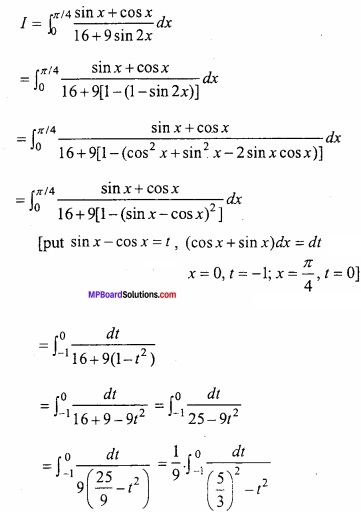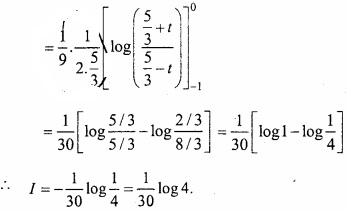# MP Board Class 12th Maths Important Questions Chapter 7B Definite Integral

## MP Board Class 12th Maths Important Questions Chapter 7B Definite Integral

### Definite Integral Important Questions

Definite Integral Long Answer Type Questions – II

Question 1.
Evaluate $$\int_{0}^{4 a} \frac{f(x)}{f(x)+f(4 a-x)} d x$$?
Solution:
Let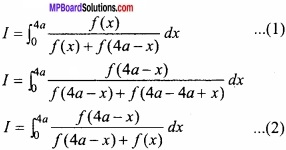Adding eqns.(1) and (2),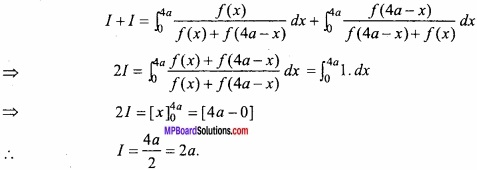Question 2.
Prove that $$\int_{\pi / 6}^{\pi / 3} \frac{d x}{1+\sqrt{\tan x}}=\frac{\pi}{12}$$? (NCERT)
Solution: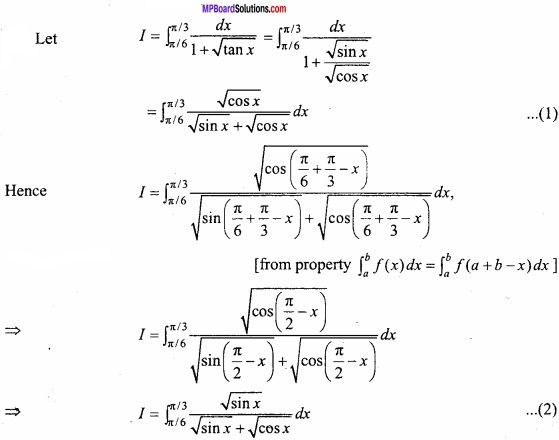Adding eqns.(1) and (2),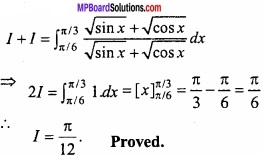Question 3.
Evaluate $$\int_{\frac{\pi}{4}}^{\frac{\pi}{2}} e^{x}(\log \sin x+\cot x) d x$$?
Solution: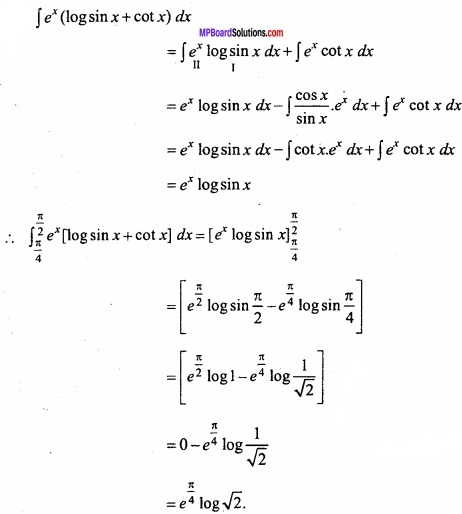Question 4.
Evaluate $$\int_{-\frac{\pi}{2}}^{\frac{\pi}{2}}|2 \sin | x|+\cos | x|| d x$$?
Solution:
Let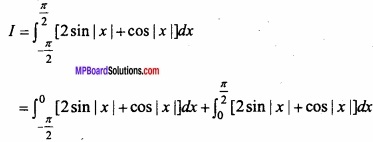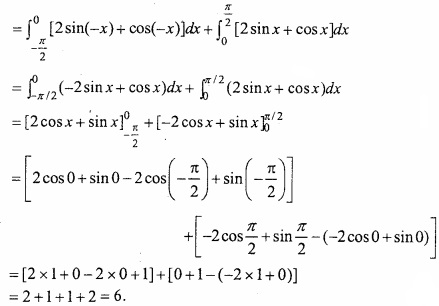Question 5.
Prove that:
$$\int_{0}^{\pi} \frac{x \tan x}{\sec x+\cos x} d x=\frac{\pi^{2}}{4}$$?
Solution: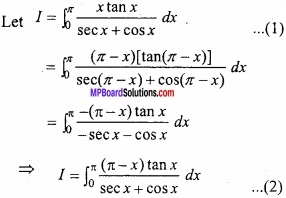Adding eqns. (1) and (2)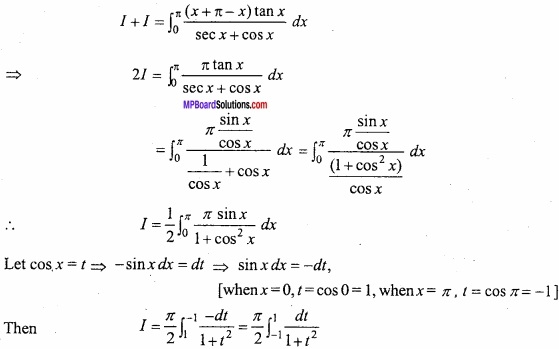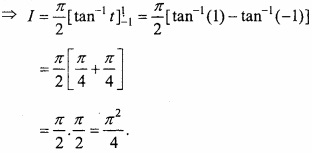Question 6.
Prove that:
$$\int_{0}^{\pi / 2} \frac{x \sin x \cos x}{\cos ^{4} x+\sin ^{4} x} d x=\frac{\pi^{2}}{16}$$?
Solution: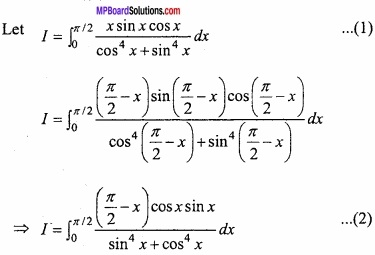Adding eqns.(1) and (2)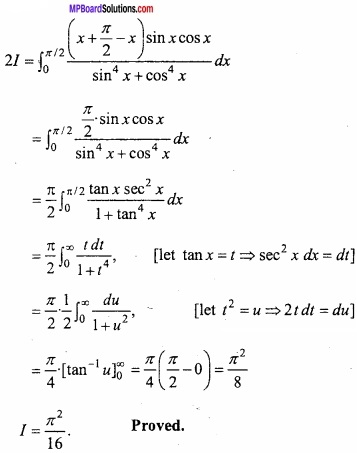Question 7.
Prove that: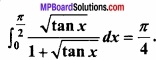Solution:
Let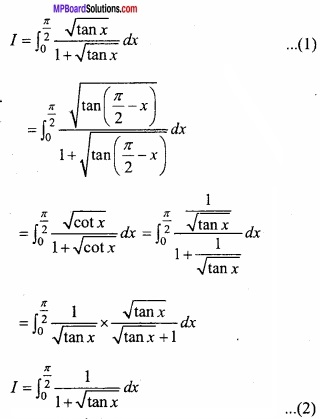Adding eqns. (1) and (2)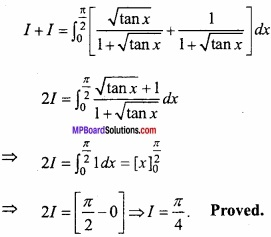Question 8.
Prove that: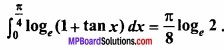Solution: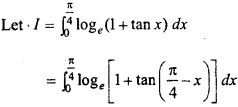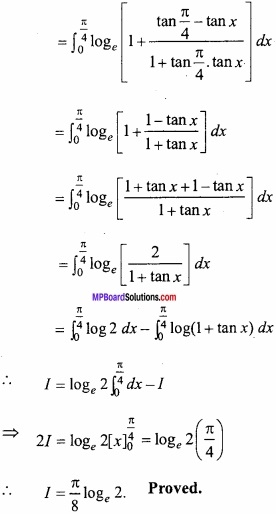Question 9.
Evaluate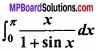Solution: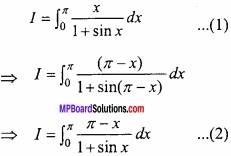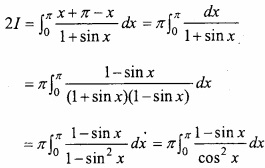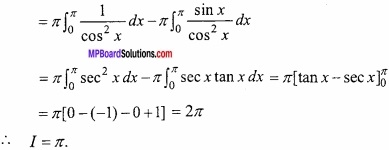Question 10.
Prove that: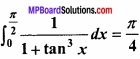Solution: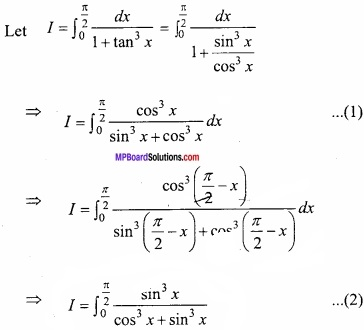Adding eqns.(1) and (2),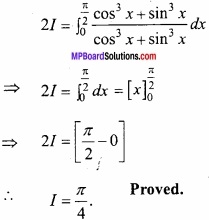Question 11.
Evaluate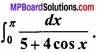Solution:
Let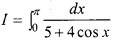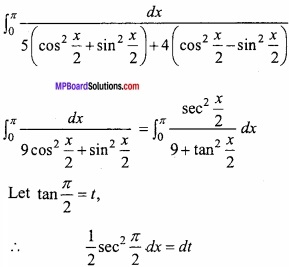When x = 0, then t = tan 0 = 0
and when x = π, then t = tan $$\frac { \pi }{ 2 }$$ = ∞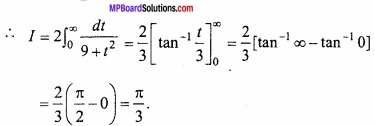Question 12.
Prove that: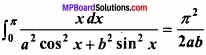Solution: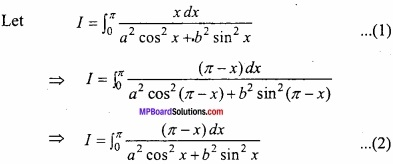Adding eqns. (1) and (2)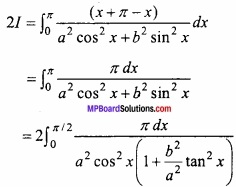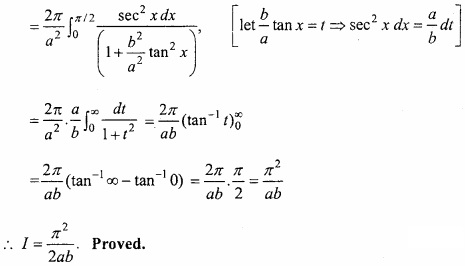Question 13.
Prove that: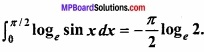Solution: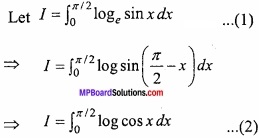Adding eqns. (1) and (2)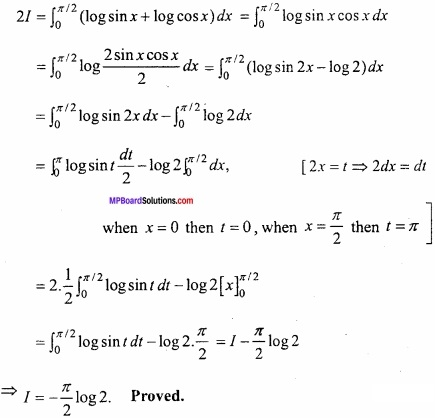Question 14.
Prove that: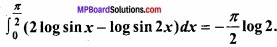Solution: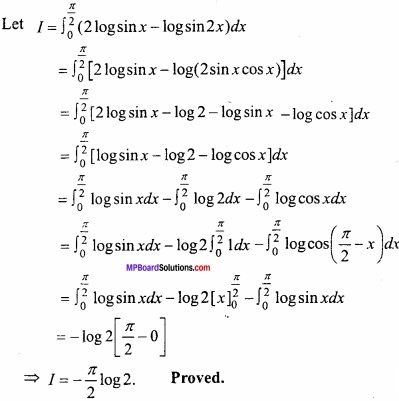Question 15.
Evaluate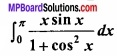Solution: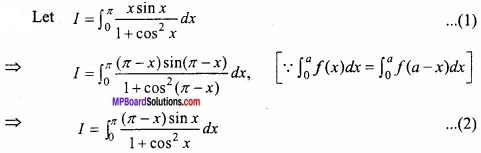Adding eqns.(1) and (2)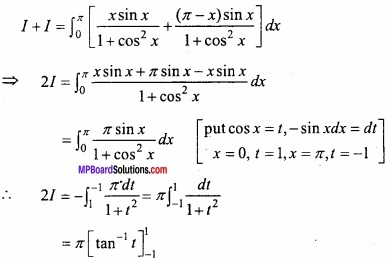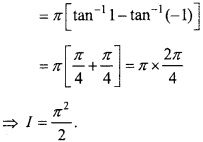Question 16.
Evaluate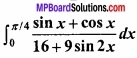Solution:
Let